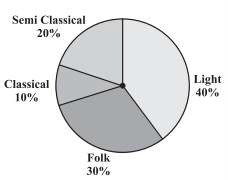# A survey was made to find the type of music that a certain group of young people liked in a city. Adjoining pie chart shows the findings of this survey. From this pie chart, answer the following: (i) If 20 people liked classical music, how many young people were surveyed? (ii) Which type of music is liked by the maximum number of people? (iii) If a cassette company were to make 1000 CD’s, how many of each type would they make?Solution

(i)

Given, 10% of x = 20

(10/100) * x = 20

x = (20 * 100) / 10 = 200

So, 200 young people were surveyed.

(ii) Since 40% of the total people surveyed liked light music and no other form of song liked more than that, we can conclude that Light music is liked by the maximum number of people.

(iii)

Classical music CDs = 10% of 1000

= (10 / 100) * 1000

= 100 CDs

Semi Classical music CDs = 20% of 1000

= (20 / 100) * 1000

= 200 CDs

Folk music CDs = 30% of 1000

= (30 / 100) * 1000

= 300 CDs

Light music CDs = 40% of 1000

= (40 / 100) * 1000

= 400 CDs(19)(1)